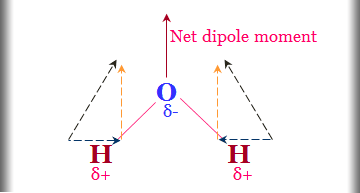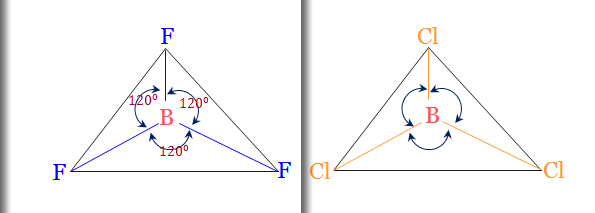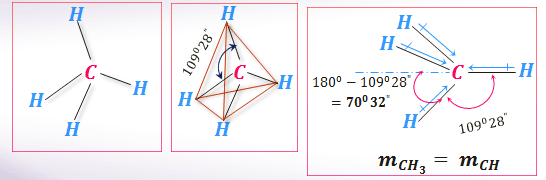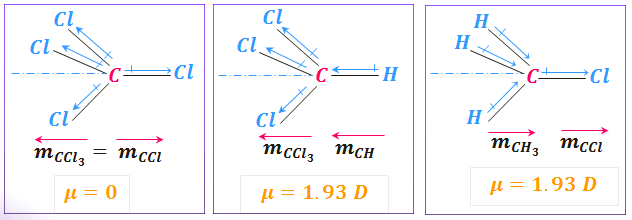# Application of Dipole Moment

### Covalent and ionic bond and application of dipole moment

Let us consider compound AB having the dipole moment μobs and the bond length l cm. If the shared electron pair lies at the midpoint of the atoms, the bond will be purely covalent bond and the present of ionic character zero.

For ionic bond, B more electronegative than A. A and B will carry a unit negative charge and uni-positive charged respectively. Thus the polarity of bond arises in the molecule AB.

∴ μionic = e × ℓ
= (4.8 × 10⁻¹⁰) ℓ esu cm.

But the dipole moment of AB not greater than zero. Thus we can calculate the percentage of ionic character of the bond.The ionic character of the bond
Question
The dipole moment of hydrochloric acid 1.03 Debye and bond length is 1.27 A. (a) Calculate the charge on the constituent atom. (b) What is the percentage of the ionic character of the bond?

Charge on the constituent atom = 0.8 × 10⁻¹⁰ esu.
Percentage of the ionic character of the bond = 16.89%.

### Dipole moment from the polarization of bond

The induced polarization
Pi = (4/3) π N₀ αi.

For gas molecules,
Pi = {(D₀ - 1)/(D₀ + 2)} M/ρ
D൦ close to unity under this condition.

{(D0 - 1)/3} × 22400 = ( 4/3 ) π N₀ r³

At NTP, M/ρ = molar volume = 22400cc/mole.
For spherical molecule, αi= r³.

or, r³ = (22400/4πN₀ ) (D₀ - 1)
= 2.94 ×10⁻²¹ (D₀ - 1)
The radius of the molecule r can be determined by measuring D൦ of the substance at NTP.

#### Mono-atomic inert gases

Mono-atomic inert gases are non-polar, and it indicates the symmetrical charge distribution in the molecule.

#### Di-atomic covalent and ionic bonding molecule

The homonuclear diatomic molecules are contained a covalent bond between the atom and largely non-polar.
Nitrogen, oxygen, and chlorine are examples of such a molecule with symmetrical charge distributions. The bonding electron pair equally shared by the two bonding atoms.

Hydrogen bromide and hydrogen iodide have non zero values of dipole moment. This indicates the unsymmetrical charge distribution between two bonding atoms.

H⁺ ㄧ I⁻

Due to the difference in electronegativity of the constituent atoms in heteronuclear diatomic molecules always polar. Electron pair is not equally shared and shifted to the more electronegative atom. Hydrogen chloride, hydrogen bromide, and hydrogen iodide are examples of such types of molecules.The polarity of hydrogen halide
Question
The difference between the electronegativity of carbon and oxygen large but the dipole moments of carbon monoxide are very low - why?

The difference in electronegativity between carbon and oxygen large in carbon monoxide is very large but the dipole moment of carbon monoxide is very low. This suggested that the charge density in the oxygen atom is somehow back-donated to the carbon atom.

Which explains by forming a coordinate covalent bond directing towards carbon atom.

#### Structure of tri-atomic molecules

Carbon dioxide, barium chloride, stannous chloride have zero dipole moment indicating that the molecules have a symmetrical charge distribution between the bond.

Carbon dioxide molecule electric moment of one carbon-oxygen bond or bond polarity cancels the electric moment of the other carbon-oxygen bond.Dipole moment of CO₂ and BeCl₂
Study online for schools and college-level
The electric moment associated with the bond arising from the difference of electronegativity called the bond moment. In molecules, the vectorial addition of the bond moments gives the resultant dipole moment of the molecule.

∴ μ2 = m12 + m22 + 2m1m2Cosθ
where m₁ and m₂ are the bond moments.

Bond moments help to calculate the bond angle of the molecule. Carbon dioxide molecule has μ = 0 and m₁ = m₂.

∴ 0 = 2m²(1 + cosθ)
or, θ = 180°

#### Water, hydrogen sulfide, and sulfur dioxide

Water, hydrogen sulfide, and sulfur dioxide have μ ≠ 0 indicating that they have non-linear structures. The bond angle can be calculated from the polarity of the molecules.

#### The polarity of the water moleculePolarity of water
The dipole moment of water
μ = 1.84 D and bond moment = 1.60 D.

∴ μ² = 2 m² (1+ cosθ )
or, (1.84)² = 2 (1.60)² (1+ cosθ )
or, θ = 105°.
The contribution of non-bonding electrons towards the total dipole moment included within the bond moment.

Question
The bond angle in hydrogen sulfide = 97° and dipole moment = 0.95 D. What is the polarity of bond in hydrogen sulfide? (Cos97° = - 0.122).

The dipole moment of hydrogen sulfide = 0.95 D
and bond angle = 97°.
m = mS-H

μ² = 2 m² (1 + cosθ )
∴ (0.95)² = 2 m² (1 + cos97°)
or, 0.9025 = 2 m² (1 - 0.122)
or, m² = 0.9025/(2 × 0.878) = 0.5139
∴ m = 0.72D

Bond moment of the S - H link = 0.72 D

#### Molecular model for tetra atomic molecules

Boron trichloride, boron trifluoride are the tetratomic molecules having dipole moment zero indicating that they have regular planar structure.

Their halogen atoms are on a plane at the corner of the equilateral triangle and boron atom at the intersection of the molecules.The polarity of boron trichloride
While other types of the molecule such as NH₃, PH₃, AsH₃ are polar (μ≠0) indicating that that the molecule has a pyramidal structure in which the three hydrogen atoms are on the plane and nitrogen atom at the apex of the pyramid in ammonia.

But NF₃ has a very small dipole moment though there is a great difference of electronegativity between N and F atoms and similar structure of NH₃.

A low value of μ of NF₃ is explained by the fact that the resultant bond moment of the three N - F bonds are acting in the opposite direction to that of the lone pair placed at the N-atom. But in NH₃, the resultant bond moment is acting in the same direction as that of the lone pair electrons.Dipole Moment of NH₃ and BF₃

#### Molecular geometry of Penta-atomic molecules

Methane, carbon tetrachloride, platinum chloride are examples having zero dipole moment. This suggests that they are either regular tetrahedral or square planer. But polar molecules of this type have pyramidal structures.

#### The polarity of the methane molecule

Let us discuss the structure of methane that has regular tetrahedral structure and the angle of each H-C-H = 109°28ˊ.CH₄ molecule
The electric moment associated with a group called group moment and it depends on the arrangement of the bonds in the group and the difference of the electronegativity of the constituent atoms forming the bonds in the group.

It can be shown that the group moment of methyl group identical to the bond moment of a carbon-hydrogen bond. Two moments cancel each other resulting in zero dipole moment of the molecule in methane.
∴ mCH₃ = 3 mCH Cos(180° -109°28՛)
= 3 mCH Cos 70°32՛
= 3 mCH × (1/3)
= mCH

Dipole moment of methane
= mCH (1 + 3 Cos 109°28՛)
= mCH {1 - (3 ×(1/3)}
= 0

####CCl₄, CHCl₃, and CH₃Cl molecules The dipole moment of methyl chloride

μ² = m₁² + m₂² + 2 m₁m₂ Cosθ
But here θ = 0° hence Cosθ = 1

∴ μ² = m₁² + m₂² + 2 m₁m₂= (m₁ + m₂)²
or, μ = (m₁ + m₂)
= (mCCl + mCH)
= (1.5 D + 0.4 D)
= 1.9 D

#### Dipole moment of chloform molecule

μ = (m₁ + m₂)= (mCH + mCCl)
= (0.4 D + 1.5 D)
= 1.9 D

A similar calculation can be done for the group moment of C₂H₄, C₃H₇, C₄H₉, etc. have the same value and equal to the bond moment of carbon-hydrogen.

The identical value of the dipole moment of the homologous alcohols and saturated hydrocarbons confirms the above statement.

#### The polarity of Hexa and hepta atomic molecules

The molecules of this type are PCl₅, AsCl₅, PF₅, etc have μ =0 indicating that they have a pyramidal structure having the center of symmetry.

Hepta-atomic molecules like SF₆, XeF₆, WF₆, etc have μ =0 indicating that these have an octahedral symmetrical structure.

Study covalent and ionic bond, application of dipole moment, structure and polarity of water, sulfur dioxide, boron trichloride, methane, chloroform